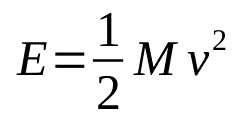# ENERGY a Physics Primer for Cyclists

## A Good Reason for the Idaho Stop!

This entry is part of 3 in the series Physics for Cyclists

As cyclists we constantly hear many people using the term energy. As with most such terms we have a general idea of what energy is, but having a general idea is not knowing and in this case knowing helps to understand why we do the things we do on our bikes.

### ENERGY a Physics Primer for Cyclists — What is energy?

Energy is the ability to do work the stuff you pay to move you and your bike. Energy comes in many forms, but this article concentrates on mechanical energy. Mechanical energy is the energy moving objects have or need to change their motion.

We also distinguish between energy and potential energy due to gravity. You and your bike contain energy but it is potential energy. Once you and your bike start to roll downhill that potential energy converts from potential energy to mechanical energy, with the result being you are moving faster! Of course, that energy is not coming from you and that is why you do not get credit for the work or the power you and your bike are putting out.

### ENERGY a Physics Primer for Cyclists — Measuring energy

We are generally familiar with two different units of energy. The first is the dietary calorie which is actually a bundle of 1,000 calories. This is the unit used most often when we discuss energy contained in food and the energy we expend when we exercise. A calorie is the amount of energy needed to raise the temperature of 1 cm3 of water 1° C. Heat is a different form of energy.

Another common unit of energy is the joule or the kilojoule (thousand joules). One joule is the energy required to lift a one Kg mass one meter in the air.

### ENERGY a Physics Primer for Cyclists — Calculating energy

The energy calculation most applicable to us as cyclists is this one:The above is the formula is the one we use to calculate our energy while cycling. E is the resulting energy, M is the combined mass of ourselves and our bike, and V is the speed. The best units to use in this formula are metric units. So find out how much you and your bike weighs in kilograms and then determine your velocity in meters per second (simply multiply your miles per hour by 0.44704), put the numbers in and that will tell you how much energy it will take to achieve that speed or how much energy you have at the given speed.

I weigh 91 Kg and the Dire Wolf weights 10 kg for a total of 101 Kg. My average speed on my last ride (as of this writing) was 21.6 mph or 9.7 meters per second. So our formula is now:

Which computes to 4,751 joules, remember, this is an average which means at times my energy was greater and at times it was less.

### ENERGY a Physics Primer for Cyclists — The Idaho stop

Now let us perform a little thought experiment. Let us say I’m tootling along at 1 m/s (a very slow 2.2 miles per hour) and I want to double my speed to 2 m/s (a still slow 4.4 mph). How much more energy do I have to expend to double the speed from 1 m/s to 2 m/s?

How much more? 2 times the energy? 4 times the energy? 8 times the energy? 10 times the energy? The answer is we need to supply four times the energy, because energy is dependent on the SQUARE of the speed. This is one reason why we are so concerned about preserving speed! The need to rebuild speed costs us both in terms of our stored energy and our fast twitch muscle (white meat) availability. In short, it is cheaper to maintain a speed than it is to have to build it back up.

To preserve that speed (ie energy) you need skills and experience. The ability to corner at a high speed is a very important skill, if you can not corner at speed you will be wasting energy. If you overreact to a gap you end up wasting energy by braking. However, it is worse to under-react to a gap because then you are without a draft and you will most likely have to go faster to get back on.

This saving of our energy is the big reason we are loathe to stop and why we lobby for the Idaho Stop! Automobiles are affected by the same physics and the solution for them is the roundabout. At roundabouts we only stop when we need to, yes cars have to slow down to navigate them, but that is much better than requiring ten cars to stop to allow one car through, when the one car could have navigated safely ten seconds earlier.

So the next time someone tells you cyclists “need to obey the laws” tell them we are doing so, the laws of physics and suggest they lobby for more roundabouts!